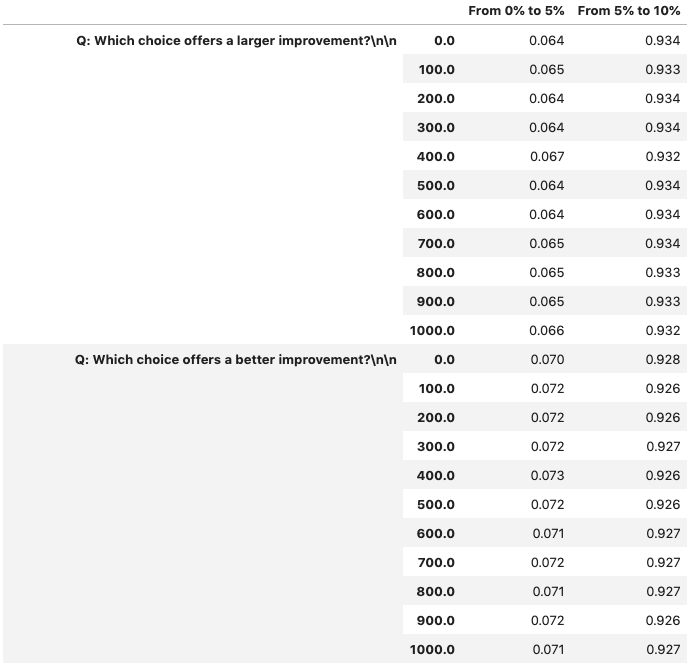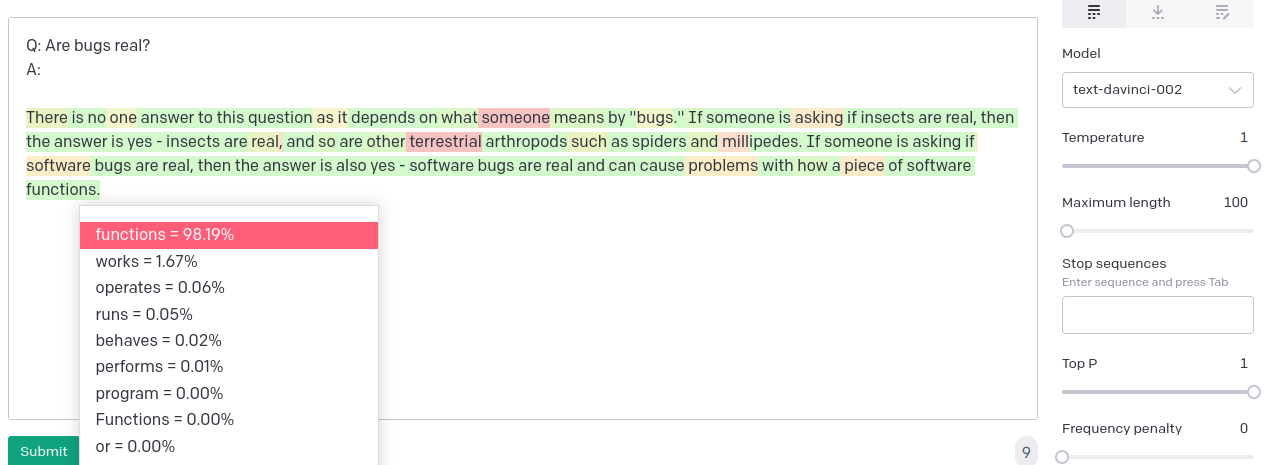# 8

The setup and motivation
There are plenty of examples in which GPT-3 has made 'obviously' bad decisions. Here is another simple game that leads to an unexpected decision when it comes to a question of choice.

Consider a game where we flip two distinct, unfair coins. The reward is \$10 for each
head we get. If it's tails, we get \$0. In this setup, let's assume that

• Coin A has a 0% probability of scoring heads.
• Coin B has a 5% probability of scoring heads.

Suppose we have a choice to bump the probability of either coin A or B getting heads by 5%. What do coin do we chose to bump? Mathematically, the choice of coin to bump shouldn't matter, since the expected value of the game increases by the same amount.

The question

Inspired by this game, I asked GPT-3 several variants of the question below, where we choose the better amongst two 'improvement' scenarios. I expected that it would approach either choice with a 50% probability.

Q: Which choice offers a better improvement?
- Option F: A probability increase from 0% to 5%, to win x dollars
- Option J: A probability increase from 5% to 10%, to win x dollars.

In general, I also expect that humans choose Option F, since the marginal increase from an impossible scenario 'feels' larger than from an unlikely scenario. This is related to the probability overweighting effect as described by Kahneman in 'Thinking Fast and Slow'.

Data

The probabilities assigned to each choice are shown below. The numbers on the second column from the left denote the level of x, and the unbolded numbers denote the probabilities.Results

• It looks like GPT-3 seems to strongly favour the latter choice, to bump the probability from 5% to 10%, for different (seemingly all) values of x. This is weird, and an unexpected result.
• What's weird is the level of conviction of choice J -  above 90%. I have no idea why this happens.
• It could be a lack of understanding of the word 'improvement'.
• The result seems robust to multiple wordings of the question.

Details

# 8

New Comment

Is this text-davinci-002 or davinci?

text-davinci-002, updated with a link to github

text-davinci-002

What's weird is the level of conviction of choice J - above 90%. I have no idea why this happens.

text-davinci-002 is often extremely confident about its "predictions" for no apparent good reason (e.g. when generating "open-ended" text being ~99% confident about the exact phrasing)This is almost certainly due to the RLHF "Instruct" tuning text-davinci-002 has been subjected to. To whatever extent probabilities output by models trained with pure SSL can be assigned an epistemic interpretation (the model's credence for the next token in a hypothetical training sample), that interpretation no longer holds for models modified by RLHF.